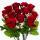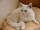# Divide money 2

Ben and Dan had the same amount of money at the start. When Ben gave 300 to Dan, the ratio of Ben 's money to Dan's money became 2:3. How much money did each have at first?

Result

x =  1500

#### Solution:

3(x-300) = 2(x+300)

x = 1500

x = 1500

Calculated by our simple equation calculator.

Leave us a comment of example and its solution (i.e. if it is still somewhat unclear...):

Showing 0 comments:Be the first to comment!#### To solve this example are needed these knowledge from mathematics:

Do you have a linear equation or system of equations and looking for its solution? Or do you have quadratic equation?

## Next similar examples:

1. Money 6Lita had some money. She spent 1/2 of it on a hand bag and 1/3 of the remainder of the blouse. She had 260.00. How much money did she have at start?
2. 7 rosesPeter buys 7 roses. When he pay for it left him 4 euro. If he bought 5 roses left him 40 euros. How many euros had Peter before buying?
3. CatOne-fifth of the monthly pocket money contributes Maros for food for his cat, half of the rest postpone for a new smartphone. The remaining € 8 spend. How much pocket money gets Maros a month?
4. Unknown number 24f we add 20, we get 50% of its triple. What is this unknown number?
5. Two machinesPerformances of two machines are in a ratio of 7:12. A machine with less power produced 406 pieces of products per shift. a) How many pieces produced per shift second machine? b) How many pieces produced two machines together for five shifts?
6. Simple equationSolve for x: 3(x + 2) = x - 18
7. Unknown number 11That number increased by three equals three times itself?
8. Unknown numberIdentify unknown number which 1/5 is 40 greater than one tenth of that number.
9. ExpressionSolve for a specified variable: P=a+4b+3c, for a
10. CakesGrandmother baked cakes. Half of its was poppy, quarter with plum jam and 16 cheesecakes. How many cakes she baked in total?
11. AlleyAlley measured a meters. At the beginning and end are planted poplar. How many we must plant poplars to get the distance between the poplars 15 meters?
12. Simple equationSolve the following simple equation: 2. (4x + 3) = 2-5. (1-x)
13. Negative in equation2x + 3 + 7x = – 24, what is the value of x?
14. SimplifySimplify the following problem and express as a decimal: 5.68-[5-(2.69+5.65-3.89) /0.5]
15. Equation 29Solve next equation: 2 ( 2x + 3 ) = 8 ( 1 - x) -5 ( x -2 )
16. Simplify 2Simplify expression: 5ab-7+3ba-9
17. HotelThe hotel has a p floors each floor has i rooms from which the third are single and the others are double. Represents the number of beds in hotel.